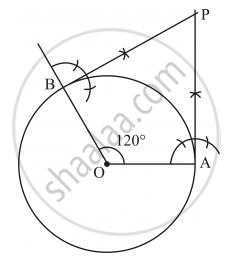Share

# Draw a Pair of Tangents to a Circle of Radius 3 Cm, Which Are Inclined to Each Other at an Angle of 60°. - Mathematics

Course

#### Question

Draw a pair of tangents to a circle of radius 3 cm, which are inclined to each other at an angle of 60°.

#### Solution

The tangents can be constructed in the following manner:

(i) Draw a circle of radius 3 cm with the centre as O.

(ii) Take a point A on the circumference of the circle and join OA. Draw a perpendicular to OA at point A.

(iii) Draw a radius OB, making an angle of 120° [(180° − 60°)] with OA.

(iv) Draw a perpendicular to OB at point B. Let both the perpendiculars intersect at point P.

PA and PB are the required tangents at an angle of 60°.Is there an error in this question or solution?